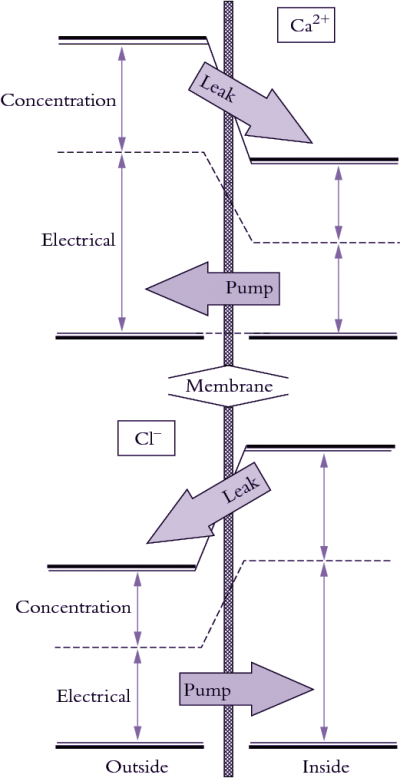# 3.6.2 - Chemical potential

Diffusion of neutral molecules at low concentrations is driven by differences in concentrations across membranes, as explained above. There are other forces that may influence solute diffusion, including the voltage gradient when considering movement of charged molecules (ions) and the hydrostatic pressure when considering movement of highly concentrated molecules (such as water in solutions). These forces can be added to give the total potential energy of a particular molecule ($\mu_s$) relative to a reference value ($\mu_s^*$):

$\mu_s = \mu_s^* + RT\ln C_s + z_s FE + V_sP \tag{16}$

Gravitational potential energy could also be added to this equation if we were to examine the total potential over a substantial height difference, but for movement of molecules across membranes this is not relevant.

The concentration term ($RT\ln C_s$) is a measure of the effect on chemical potential of the concentration of solutes (actually the activity of the solutes which is usually somewhat less than total concentration). The gas constant, $R$ (8.314 joules mol–1K–1), and absolute temperature, $T$ (in degrees Kelvin, which is equals 273 plus temperature in degrees Celsius), account for the effects of temperature on chemical potential.

Incidentally, from this term the well-known van‘t Hoff relation is derived for osmotic pressure $\pi$, as given at the beginning of this chapter:

$\pi = RTC \tag{1}$

## 3.0-Ch-Fig-3.69.pngFigure 3.69. Illustration of how electrical and concentration terms contribute to electrochemical potential of ions. Calcium (top) commonly tends to leak into cells and must be pumped out whereas chloride tends to leak out and must be pumped in to be accumulated.

where $R$ is the gas constant (8.31 joules mol–1K–1), $T$ is the absolute temperature in degrees Kelvin (273 plus degrees Celsius), and $C$ is the solute concentration (Osmoles L-1). At 25 ºC, $RT$ equals 2.48 liter-MPa per mole, and ($\pi$) is in units of MPa. Hence a concentration of 200 mOsmoles L-1 has an osmotic pressure of 0.5 MPa.

The electrical term ($z_s FE$ ) is a measure of the effect of voltage ($E$ ) on chemical potential. The charge on a solute ($z$) determines whether an ion is repelled or attracted by a particular voltage. Electrical charge and concentration are related by the Faraday constant ($F$ ) which is 96,490 coulombs mol–1. The electrical and concentration terms form the basis of the Nernst equation (see below).

The pressure term measures the effect of hydrostatic pressure on chemical potential, where $P = \text{Pressure}$ and $\overline{V}_s$ is the partial molar volume of the solute.

Molecules diffuse across a membrane down a chemical potential gradient, that is, from higher to lower chemical potential. Diffusion continues until the difference in chemical potential equals zero, when equilibrium is reached. The direction of a chemical potential gradient relative to transport of a molecule across that membrane is important because it indicates whether energy is or is not added to make transport proceed (Figure 3.69). Osmotic ‘engines’ must actively pump solutes against a chemical potential gradient across membranes to generate $P$ in a cell. Sometimes ions move against a concentration gradient even when the flux is entirely passive (no energy input) because the voltage term dominates the concentration term in Equation 16. In this case, ions flow according to gradients in electrical and total chemical potential. For this reason, the chemical potential of ions is best referred to as the electrochemical potential.